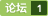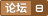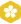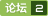### #define STREQ(a,b) (*(a)==*(b)&&strcmp((a),(b))==0) [问题点数：20分，结帖人blueskyrabbit]#define max(a,b) ((a) > (b)) ? (a) : (b)的问题
[code=C/C++]rn#include rn#<em>define</em> max(a,b) ((a)>(b))?(a):(b)rn//sockfd=3,fileno(stdin)=0;rnmaxfdp1=1+max(sockfd,fileno(stdin));//如果这样maxfdp1=3;rnmaxfdp1=1+(max(sockfd,fileno(stdin)));//如果这样maxfdp1=4;rnmaxfdp1=1+max(3,0);//maxfdp1=4;rn[/code]rn为什么第一次调用会出现这种问题？在宏调用两边加个括号就没事了，而第三次则不用？
#define max(a,b) ((a) > (b) ? (a) : (b))的问题
int a = 5, b = 0;rnmax(++a, b);// a 的值增加了2次rnmax(++a, b+10); // a 的值只增加了1次rn别人的文章中看到的，增加几次是怎么发生的，应怎么理解啊？rn请老师指导，不胜感谢！rnrn
#define macro(a,b) (a^=b^=a^=b);的原理
#<em>define</em> macro(a,b) (a^=b^=a^=b);rnrn char a='a';rn char c='b';rn macro(a,c);rn //实现了a与c的互换rn我想问大家这个宏的原理是什么，能给小弟解释一下么，谢谢！
#define Mid(a,b,c) (a+b+c) - ((a>b?a:b)>c?(a>b?a:b):c)-((a

POJ 2406 KMP 解题报告
Power StringsDescriptionGiven two strings a and b we <em>define</em> a*b to be their concatenation. For example, if a = “abc” and b = “def” then a*b = “abcdef”. If we think of concatenation as multiplication, e
Power Strings
-
（POJ2406）Power Strings
Power Strings DescriptionGiven two strings a and b we <em>define</em> a*b to be their concatenation. For example, if a = “abc” and b = “def” then a*b = “abcdef”. If we think of concatenation as multiplication,
UVA10298 Power Strings
Given two strings a and b we deﬁne a∗b to be their concatenation. For example, if a = ‘abc’ and b = ‘def’ then a∗b = ‘abcdef’. If we think of concatenation as multiplication, exponentiation by a non-n...
KMP之Power Strings
Power Strings   Given two strings a and b we <em>define</em> a*b to be their concatenation. For example, if a = "abc" and b = "def" then a*b = "abcdef". If we think of concatenation as multiplication,
A^B == B^A?
Solve the equation in the name of this problem.nnInputnnThere are several cases. Each of them has a single real number as the smaller one of a and b in the equation on a separate line. You may assume it's no smaller than 1.1. Proceed until the end of file.nnOutputnnFor each case, print the other number on a single line. Keep 5 digits after decimal point. If no such number exists, print -1.nnSample Inputnn2n10nSample Outputnn4.00000n-1
a,b 和（a,b）
main()rnrn int a, b,c, d;rn a=3;rn b=5;rn c=a,b;rn d=(a,b);rnrn printf("c=%d" ,c);rn printf("d=%d" ,d);rnrnrnrn？
A>B?A:B

a^=b^=a^=b

#define a b 意味着什么
b 是一个类其实现也有； a也是类，但是没看到具体实现，只有头文件。
Power Strings
Power Strings Time Limit: 1000MS Memory limit: 65536K 题目描述 Given two strings a and b we <em>define</em> a*b to be their concatenation. For example, if a = "abc" and b = "def" then a*b = "abcdef".
POJ2406:Power Strings(后缀数组DC3)
Description Given two strings a and b we <em>define</em> a*b to be their concatenation. For example, if a = "abc" and b = "def" then a*b = "abcdef". If we think of concatenation as multiplication, exponenti
poj 2406 最小循环节kmp
Given two strings a and b we <em>define</em> a*b to be their concatenation. For example, if a = “abc” and b = “def” then a*b = “abcdef”. If we think of concatenation as multiplication, exponentiation by a non-n
#define pin (int*);pin a,b; a,b 类型不同？？
#<em>define</em> pin (int*);rnpin a,b;rn本意是a和b都是int型指针，但是实际上变成int* a,b;rna是int型指针，而b是int型变量。rn这是应该使用typedef来代替<em>define</em>，这样a和b就都是int型指针了。rn
#define DIV(a,b) a/b表示什么意思？
#<em>define</em> DIV(a,b) a/b表示什么意思？

A?B:C 等价于 if(A)     B; else    C; Problem H: C语言实验——整数位 Description 输入一个不多于5位的正整数，要求： （1）求出它是几位数； （2）分别输出每一位数字； （3）按逆序输出各位数字。 Input 输入一个不多于5位的正整数。 Output 输出数据有3行，第一行为正整数位数，第二行为各位数字，
((A*)&b)和((A)B)的区别
class Arn rn ...rn public:rn void fun()rn rn hello();rn rn virtual void hello...rn ...rnrnrnclass B:public Arn rn ...rn virtual void hello()...rn ...rnrnrnmain()rnrn B b;rn ((A*)&b)->hello();rn ((A)b).hello();rnrnrn请问((A*)&b)和((A)b)有何本质的区别啊？谢谢rn
a = b + (b = a) * 0;
[code=C#]int a = 10, b = 21;rn a = b + (b = a) * 0;rn Console.WriteLine("a=0,b=1",a,b);[/code]rn这段代码为什么可以把a，b的值互换？rnrn a = b + (b = a) * 0;中选计算括号里的b = a，在计算(b = a) * 0，到b + (b = a) * 0的时候最左边的b已经是a的值了，a应该还是原来a的值才对啊。难道是在计算表达式b + (b = a) * 0中，(b = a)根本没有真正互换，直到表达式结束后才互换的？rnrnC++中输出确实是a==b==10的，c#中就互换了
G - Power Strings POJ 2406 （字符串的周期）
G - Power Strings Time Limit:3000MS Memory Limit:65536KB 64bit IO Format:%I64d & %I64u Submit Status Practice POJ 2406 Description Given two strings a and b we <em>define</em> a*b to be their concatenati
#define A(...) B 表示什么意思呀？

POJ 2406 Power Strings
kmp
a, b = b, a + b,运行机制
rt，按c语言的话，必须使用一个临时变量rn[code=c]rntmp = a + brn a = brn b = tmprn[/code]rnpython中如何运行，求解释
Inc(A, B) PK A := A + B

a += b 和 a = a + b有区别？

/*设计一个程序，要求用带参数的宏交换两个参数的值，在程序中输入两个整数并将它们交换，然后输出交换后的值。*/rn#include rn#<em>define</em> Exchange(a,b,c) c=a;a=b;b=c;rnrnvoid main()rnrnrn/*定义三个整数*/rnint A1,A2,m;rnrn/*从键盘输入A1，A2的值*/rnprintf("请输入A1的值：\n"); rnscanf("%d",&A1); rn/*输入A2的值*/ rnprintf("请输入实数A2的值：\n"); rnscanf("%d",&A2); rn/*交换A1、A2的值*/rnExchange(A1,A2,m);rn/*打印A1、A2的值*/rnprintf("A1=%d,A2=%d\n",A1,A2);rnrnrn这是源代码 我知道 宏但Exchange(a,b,c) c=a;a=b;b=c; 什么意思
【求助】关于a&＝b a>>＝b a<<＝b a^=b a|=b 的意思
【求助】关于a&＝b a>>＝b a<>＝b a<<＝b a^=b a|=brnrn这5个比较陌生，不知道啥意思rn
F - Power Strings （类型4：求最小循环节有几个）
Given two strings a and b we <em>define</em> a*b to be their concatenation. For example, if a = &quot;abc&quot; and b = &quot;def&quot; then a*b = &quot;abcdef&quot;. If we think of concatenation as multiplication, exponentiation by a non-...
POJ 2406 - Power String
Description Given two strings a and b we <em>define</em> a*b to be their concatenation. For example, if a = "abc" and b = "def" then a*b = "abcdef". If we think of concatenation as multiplication, exponentiat
E - Power Strings POJ - 2406 (后缀数组｜｜ｋｍｐ）

b b1;//a(),b(),~b(),~a()为什么不是a(),b(),~a()，~b()呢?
[code=C/C++]rnclass arnrnpublic:rn a()cout<<
A a,　b;a = b;与 A a;A b = a; 有什么不同？
A a,　b;a = b;与 A a;A b = a; 有什么不同？
A^B(A的B次方)——人见人爱A^B，Rightmost Digit 。

a=b; b=c;

b = (++a)+(++a);
#include rnvoid main ( ) rn rnint a =8;rnint b =0;rnb = (++a)+(++a);rnprintf ( "%d\n",b) ; rn rn rn竟然等于20！怎么算的？
poj2406 Power Strings-------KMP
Power Strings   Time Limit:3000MS Memory Limit:65536K Total Submissions:23083 Accepted:9679 Description Given two strings a and b we <em>define</em> a*b to be
poj2406 Power Strings kmp

Power Strings （KMP）
#include&amp;lt;stdio.h&amp;gt; #include &amp;lt;string.h&amp;gt; #<em>define</em> maxn 1000010 char a[maxn]; int nxt[maxn]; int len; void getnxt() { int i=1,j=0; nxt=0; while(i&amp;lt;len) { if(a[i]==a[j]) { ...
poj2405 Power Strings KMP
Power Strings   Given two strings a and b we <em>define</em> a*b to be their concatenation. For example, if a = &quot;abc&quot; and b = &quot;def&quot; then a*b = &quot;abcdef&quot;. If we think of concatenation as multiplication, expone...
【UVa】10298 - Power Strings
Problem hereGiven two strings a and b we <em>define</em> a ∗ b to be their concatenation. For example, if a = ‘abc’ and b = ‘def’ then a ∗ b = ‘abcdef’. If we think of concatenation as multiplication, exponen

A - 人见人爱A^B
A - 人见人爱A^B Time Limit:1000MS     Memory Limit:32768KB     64bit IO Format:%I64d & %I64u Submit Status Description 求A^B的最后三位数表示的整数。  说明：A^B的含义是“A的B次方”  Input 输入数据包含多个测
A a=b;的问题?

c=(a==b)?a:b;和if(a==b) c=a; else c=b;那个快？
c=(a==b)?a:b;和if(a==b) c=a; else c=b;那个快？
B继承于A，A a=new B()与B a=new B()区别

Power Strings@POJ 2406
Given two strings a and b we <em>define</em> a*b to be their concatenation. For example, if a = &quot;abc&quot; and b = &quot;def&quot; then a*b = &quot;abcdef&quot;. If we think of concatenation as multiplication, exponentiation by a non...
poj 2406 KMP
http://poj.org/problem?id=2406
FZU 1011 Power Strings（KMP匹配算法）
Problem 1011 Power Strings Accept: 914 Submit: 2751 Time Limit: 1000 mSec Memory Limit : 32768 KBProblem DescriptionGiven two strings a and b we <em>define</em> a*b to be their concatenation. For exampl
POJ2406之后缀数组
E - Power StringsDescription Given two strings a and b we <em>define</em> a*b to be their concatenation. For example, if a = “abc” and b = “def” then a*b = “abcdef”. If we think of concatenation as multiplicat
【poj2406】Power Strings——KMP

a=b, b=c, a=c??

return a>b? a:b是什么意思
public int shu(double a,double b)rnrn return a>b? a:brn
poj 2406 Power Strings KMP
Description Given two strings a and b we <em>define</em> a*b to be their concatenation. For example, if a = "abc" and b = "def" then a*b = "abcdef". If we think of concatenation as multiplication, exponentiat

Python心法：a,b=b,a原理

import java.util.Scanner; public class Homework4 { public static void main(String[] args) { // 输入两个数求次幂方:如：3 3 27 System.out.println(&quot;输入两个数：&quot;); Scanner scan = new Scanner(System.in); int po...
/*+ use_hash(a,b) full(a) full(b)*/
select /*+ use_hash(a,b) full(a) full(b)*/*rn from A, Brnwhere .............rnrn请问下/*+ use_hash(a,b) full(a) full(b)*/ 这种连接什么适合什么时候用? 具体原理是什么? 谢谢
a=b b=c 就a=c?
Integer a=new Integer(3);rnInteger b=new Integer(3);rnSystem.out.println(a==3);rnSystem.out.println(b==3);rnSystem.out.println(a==b);

'a'-'A'=='b'-'B'为什么是false？？
‘a'代表97，‘A’代表65rnrn搞不懂 非常简单的问题，。。

a^b

a=b
#include rnrnusing namespace std;rnrnclass C1rnrnpublic:rn int g;rn void Init(int g)rn rn this->g=g;rn rn void print()rn rn int t;rn cout<>t;rn rn;rnrnvoid main()rnrn C1 a,b;rn b.Init(16);rn [b]a=b;[/b] a.print(); rnrnrnrnrn就上面一段程序,a=b,突然有一种陌生感,请教,谢谢。
a b
a=1rnb=2rna=b+(b=a)*0rna=?rnb=?
A∩B

(a*|b*)* 与(a*b*)* 有什么区别？
(a*|b*)* 与(a*b*)* 有什么区别？rnrn
max=(a>b)?a:b;用法困惑

a b c怎么会成为a b
main()rnrn char a,b,c;rn scanf("%c%c%c",&a,&b,&c);rn printf("%c%c%c",a,b,c);rn rn输入a b c，输出的应该是a b c，可竟然是a b 为何？rn就是下面这样的rna b crna b
a!B

python中==和is的区别，a==b和a is b

*a ^= *b ^= *a ^= *b是什么意思
void swap(int *a, int *b)rnrn *a ^= *b ^= *a ^= *b;rnrnrn^=啥意思啊 还有整个过程是怎么样的 求大牛解释~~~ 先谢谢了！

#define a b c 是什么意思
#<em>define</em> DECLARE_SERIAL(class_name) \rn DECLARE_DYNCREATE(class_name) \rn friend CArchive& AFXAPI operator>>(CArchive& ar, class_name* &pOb);rn这个定义的什么

public class Swaprn public static void main(String [] args)rn int a = 1,b = 2;rn a^=b^(b^=a^b);rn System.out.println(a+" "+b);rn rnrnrn输出： 2 1rnrn若int x = b^(b^=a^b)输出 x值为3，我想知道括号内(b^=a^b)表达式值是什么？rnrn如果按^优先级高于^=，执行b^=a^b后，b值为1，而最后a值为2，那么表达式（b^=a^b)应当是3，可是这里面的原理是什么呢？
if(a>b>c) 先执行a>b 还是 b>c ????
#include rnrnvoid main()rnrnint a=5,b=4,c=3,d=2;rnif(a>b>c)rnprintf("%d\n",d);rnelse if((c-1>=d)==1)rnprintf("%d\n",d+1);rnelsernprintf("%d\n",d+2);rn
int a=1,b;b=a++;求a和b
int a=1,b;b=a++;求a和b
【新手问题】A是B的父类，A a=new B()
public abstract class A rn rn public A() rn rn Console.WriteLine( 'A '); rn rn public virtual void Fun() rn rn Console.WriteLine( "A.Fun() "); rn rn rnpublic class B: A rn rn public B() rn rn Console.WriteLine( 'B '); rn rn public new void Fun() rn rn Console.WriteLine( "B.Fun() "); rn rn public static void Main() rn rn A a = new B(); rn a.Fun(); rn rn rnrnrn执行结果如下： rnA rnB rnA.Fun() rnrnrnrn如果把class B中的Fun（）的new改成override,执行结果如下： rnA rnB rnB.Fun() rnrnrnrn问题①：A a=new B()和B a=new B()具体有什么区别？rn问题②：我知道如果是B a=new B()的话，那无论class B中Fun（）是new还是override，最后输出的都会是B.Fun(),为什么换成A a=new B()就不同了？（问题还是在于A a=new B()）

Python中a += b 并不一定等价于a = a + b

PyMOL_Users_Manual下载